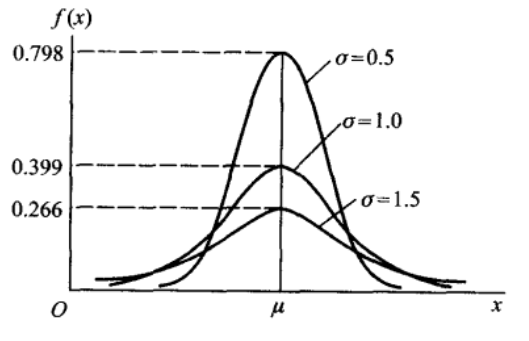离散型随机变量的分布

伯努利分布

$P(X=k) = p^k(1-p)^{1-k}, k = 0,1 (1<p<1)$

二项分布

$P(X=k) = C_n^k p^k q^{n-k}, k = 0,1,2,…,n$

$\sum_{k=1}^nP(X=k) = \sum_{k=1}^nC_n^k p^k q^{n-k} = (p+q)^n = 1$

泊松分布

$P(X=k) = \frac {\lambda^ke^{-\lambda}}{k!}, k=0,1,2….$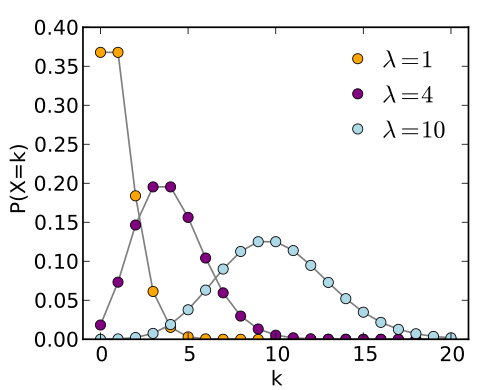$$\lim_{n \to \infty} C_n^kp^k(1-p)^{n-k} = \frac{\lambda^ke^{-\lambda}}{k!}$$

连续型随机变量的分布

分布函数和概率密度函数

$X$ 是一个随机变量，$x$ 是任意实数，则 $X$ 的分布函数定义为

$F(x) = P(X \le x), (-\infty<x<\infty)$

$P(x_1 < X \le x_2) = p(X \le x_2) - p(X \le x_1) = F(x_2) - F(x_1)$

$F(x) = \int_{-\infty}^x f(t) dt$

1. $f(x) \ge 0$

2. $\int_{-\infty}^{\infty} f(x) dx = 1$

3.对于任意实数 $x_1,x_2(x_1 < x_2)$，有$$P(x_1< X \le x_2) = F(x_2) - F(x_1) = \int_{x_1}^{x_2} f(x) dx$$

$F’(x) = f(x)$

$P(x <X \le x+\Delta x) \approx f(x)\Delta x$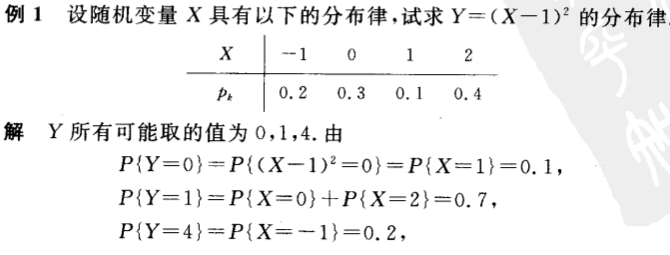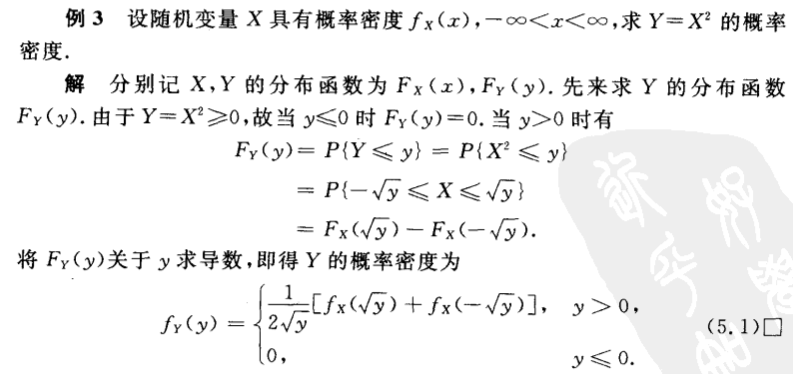均匀分布

$$f(x) = \begin{cases} \frac{1}{b-a} &{a<x<b} \\ 0&{其他}\end{cases}$$

指数分布

$$f(x) = \begin{cases} \frac{1}{\theta}e^{-x / \theta} &{x>0} \\ 0&{其他}\end{cases}$$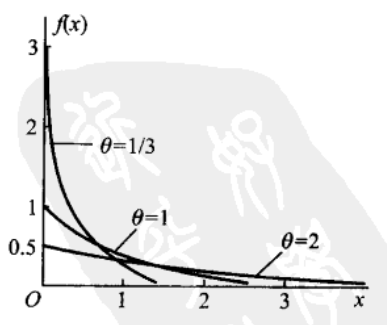$$F(x) = \begin{cases} 1-e^{-x / \theta} &{x>0} \\ 0&{其他}\end{cases}$$

$P(X>s+t | X>s) = P(X>t)$

正态分布

$$f(x) = \frac{1}{\sqrt{2\pi}\sigma}e^{-\frac{(x-\mu)^2}{2\sigma^2}}$$